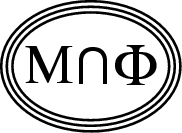Back to
OP page

Combined
List

List by
Contributors

# IMPOSSIBILITY THEOREMS IN MATHEMATICAL PHYSICS

### Abstract   It is proposed that certain common expressions such as "no exact formula exists" or "no explicit solution is expected" are to be made precise so that these assertions become mathematically provable or perhaps disprovable. Two concrete instances are suggested, one in statistical mechanics, the other in quantum mechanics.

Comment by Alan Sokal

Some of the greatest contributions to mathematics, but particularly in the 19th and 20th centuries, were impossibility theorems. Already the Ancients contributed some: For instance, the irrationality of the square root of 2 can be interpreted this way. From the 19th century we remember Abel's proof of the impossibility of solving the quintic equation by roots alone; Lindenman's proof of the transcendental nature of Pi; Beltrami's presentation of a model for hyperbolic geometry, thus proving the impossibility of "proving" Euclid's Fifth Postulate; and a less known essay of Bruns showing that in the Newtonian 3-body problem there are no constants of motion, algebraic but algebraically independent of the ten classical ones. In the 20th century, perhaps a peak of all mathematics was Godel's theorem to the effect that no consistent and "provable" axiomatization of sufficiently complex theories are possible. In this category falls also Hilbert's 10th problem, to whose solution Julia Robinson made an important contribution.

I propose to raise the challenge of coming up with and solving some such problems in the area of mathematical physics. Most of us, practitioners of this subtle art, know of sayings such as "This or that problem has no explicit solution" or "exact solution." Such things should not just be asserted; they should be proved. Of course -- as the above cited history of mathematics shows -- one must first formulate an exact definition of what it means (in any given context) an "exact solution" or any other characterization whose impossibility is asserted. Even just the very setting up of such a definition would be a worthwhile task.

Let me mention two specific problems of this type that come to my mind.

1.  A great event in statistical mechanics was Onsager's famous formula for the 2-dimensional Ising Model. Most people agree that no such "formula" exists for the 3-dimensional case.  What does "formula" mean in this context? And is the nonexistence for the 3-dimensional case a theorem?

2.  In quantum mechanics surely most of us heard it asserted that the Helium atom's energy levels cannot be determined "exactly" as those of the Hydrogen atom can.  Again, what does "exactly" mean here?   Could this be proved?